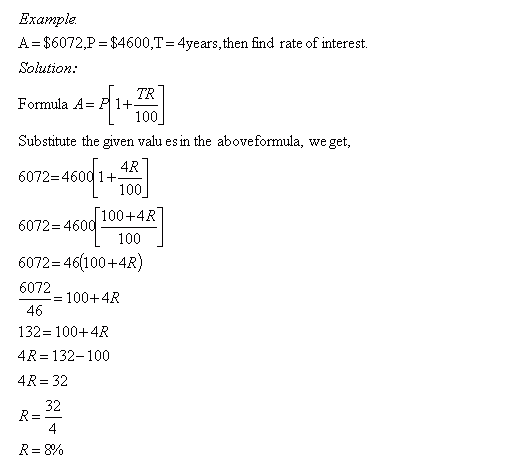Email us to get an instant 20% discount on highly effective K-12 Math & English kwizNET Programs!

#### Online Quiz (WorksheetABCD)

Questions Per Quiz = 2 4 6 8 10

### Grade 8 - Mathematics9.32 Simple Interest - VIIIDirections: Solve the following problems. Also write at least ten examples of your own.
 Q 1: A = \$5428, P = \$4600 and T = 2years, then find rate of interest.8%6%5%9% Q 2: A = \$3556, P = \$2800 and T = 3years, then find rate of interest.6%8%3%9% Q 3: A = \$16352, P = \$14600 and T = 6years, then find rate of interest.1.5%4.5%3%2% Q 4: A = \$5304, P = \$4800 and T = 3years, then find rate of interest.3.5%4.5%2%2.5% Q 5: A = \$2950, P = \$2500 and T = 3years, then find rate of interest.5.5%6%3%5% Q 6: A = \$8284, P = \$7600 and T = 3years, then find rate of interest.3%3.5%4%2.5% Question 7: This question is available to subscribers only! Question 8: This question is available to subscribers only!

#### Subscription to kwizNET Learning System offers the following benefits:

• Unrestricted access to grade appropriate lessons, quizzes, & printable worksheets
• Instant scoring of online quizzes
• Progress tracking and award certificates to keep your student motivated
• Unlimited practice with auto-generated 'WIZ MATH' quizzes